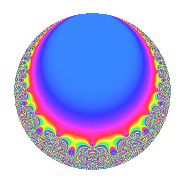# Properties

 Label 27.9.b.cLevel 27 Weight 9 Character orbit 27.b Analytic conductor 10.999 Analytic rank 0 Dimension 2 CM No Inner twists 2

# Related objects

## Newspace parameters

 Level: $$N$$ = $$27 = 3^{3}$$ Weight: $$k$$ = $$9$$ Character orbit: $$[\chi]$$ = 27.b (of order $$2$$ and degree $$1$$)

## Newform invariants

 Self dual: No Analytic conductor: $$10.9992224717$$ Analytic rank: $$0$$ Dimension: $$2$$ Coefficient field: $$\Q(\sqrt{-30})$$ Coefficient ring: $$\Z[a_1, a_2]$$ Coefficient ring index: $$3$$ Sato-Tate group: $\mathrm{SU}(2)[C_{2}]$

## $q$-expansion

Coefficients of the $$q$$-expansion are expressed in terms of $$\beta = 3\sqrt{-30}$$. We also show the integral $$q$$-expansion of the trace form.

 $$f(q)$$ $$=$$ $$q + \beta q^{2} -14 q^{4} + 26 \beta q^{5} -679 q^{7} + 242 \beta q^{8} +O(q^{10})$$ $$q + \beta q^{2} -14 q^{4} + 26 \beta q^{5} -679 q^{7} + 242 \beta q^{8} -7020 q^{10} + 814 \beta q^{11} -30817 q^{13} -679 \beta q^{14} -68924 q^{16} -7806 \beta q^{17} -138391 q^{19} -364 \beta q^{20} -219780 q^{22} + 18482 \beta q^{23} + 208105 q^{25} -30817 \beta q^{26} + 9506 q^{28} + 80740 \beta q^{29} + 352214 q^{31} -6972 \beta q^{32} + 2107620 q^{34} -17654 \beta q^{35} + 1189991 q^{37} -138391 \beta q^{38} -1698840 q^{40} -66580 \beta q^{41} + 6246086 q^{43} -11396 \beta q^{44} -4990140 q^{46} -146 \beta q^{47} -5303760 q^{49} + 208105 \beta q^{50} + 431438 q^{52} + 765468 \beta q^{53} -5714280 q^{55} -164318 \beta q^{56} -21799800 q^{58} -641206 \beta q^{59} + 16580399 q^{61} + 352214 \beta q^{62} -15762104 q^{64} -801242 \beta q^{65} + 7667153 q^{67} + 109284 \beta q^{68} + 4766580 q^{70} -1413192 \beta q^{71} + 24949631 q^{73} + 1189991 \beta q^{74} + 1937474 q^{76} -552706 \beta q^{77} + 41685089 q^{79} -1792024 \beta q^{80} + 17976600 q^{82} + 2722060 \beta q^{83} + 54798120 q^{85} + 6246086 \beta q^{86} -53186760 q^{88} -45078 \beta q^{89} + 20924743 q^{91} -258748 \beta q^{92} + 39420 q^{94} -3598166 \beta q^{95} -105926089 q^{97} -5303760 \beta q^{98} +O(q^{100})$$ $$\operatorname{Tr}(f)(q)$$ $$=$$ $$2q - 28q^{4} - 1358q^{7} + O(q^{10})$$ $$2q - 28q^{4} - 1358q^{7} - 14040q^{10} - 61634q^{13} - 137848q^{16} - 276782q^{19} - 439560q^{22} + 416210q^{25} + 19012q^{28} + 704428q^{31} + 4215240q^{34} + 2379982q^{37} - 3397680q^{40} + 12492172q^{43} - 9980280q^{46} - 10607520q^{49} + 862876q^{52} - 11428560q^{55} - 43599600q^{58} + 33160798q^{61} - 31524208q^{64} + 15334306q^{67} + 9533160q^{70} + 49899262q^{73} + 3874948q^{76} + 83370178q^{79} + 35953200q^{82} + 109596240q^{85} - 106373520q^{88} + 41849486q^{91} + 78840q^{94} - 211852178q^{97} + O(q^{100})$$

## Character Values

We give the values of $$\chi$$ on generators for $$\left(\mathbb{Z}/27\mathbb{Z}\right)^\times$$.

 $$n$$ $$2$$ $$\chi(n)$$ $$-1$$

## Embeddings

For each embedding $$\iota_m$$ of the coefficient field, the values $$\iota_m(a_n)$$ are shown below.

For more information on an embedded modular form you can click on its label.

Label $$\iota_m(\nu)$$ $$a_{2}$$ $$a_{3}$$ $$a_{4}$$ $$a_{5}$$ $$a_{6}$$ $$a_{7}$$ $$a_{8}$$ $$a_{9}$$ $$a_{10}$$
26.1
 − 5.47723i 5.47723i
16.4317i 0 −14.0000 427.224i 0 −679.000 3976.47i 0 −7020.00
26.2 16.4317i 0 −14.0000 427.224i 0 −679.000 3976.47i 0 −7020.00
 $$n$$: e.g. 2-40 or 990-1000 Significant digits: Format: Complex embeddings Normalized embeddings Satake parameters Satake angles

## Inner twists

Char. orbit Parity Mult. Self Twist Proved
1.a Even 1 trivial yes
3.b Odd 1 yes

## Hecke kernels

This newform can be constructed as the kernel of the linear operator $$T_{2}^{2} + 270$$ acting on $$S_{9}^{\mathrm{new}}(27, [\chi])$$.